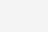# SUMPRODUCT函数写日期的三种方法=SUMPRODUCT((\$C\$2:\$C\$3001=\$G3)*1,

(\$B\$2:\$B\$3001=H\$2)*1,

(\$A\$2:\$A\$3001>=DATE(2018,3,1))*1,

(\$A\$2:\$A\$3001<=DATE(2018,3,31))*1,

\$D\$2:\$D\$3001)

=SUMIFS(\$D:\$D,\$B:\$B,H\$2,\$C:\$C,\$G3,\$A:\$A,’>=2018/3/1′,\$A:\$A,'<=2018/3/31′)

=SUMIFS(\$D:\$D,\$B:\$B,H\$2,\$C:\$C,\$G3,\$A:\$A,’>=’&\$I\$1,\$A:\$A,'<=’&\$L\$1)

(2)

3.5K

90.2K

3.3K

10.8K

2.5K